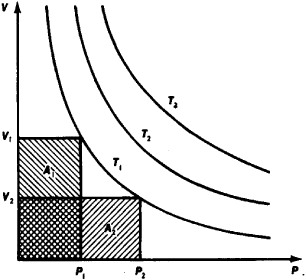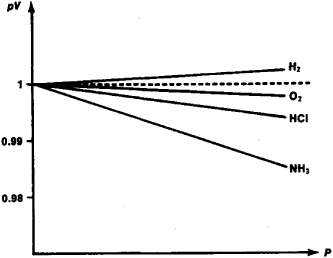# Boyle-Mariotte Law

Also found in: Dictionary, Thesaurus, Medical.
The following article is from The Great Soviet Encyclopedia (1979). It might be outdated or ideologically biased.

## Boyle-Mariotte Law

one of the fundamental gas laws, according to which, at a constant temperature, the volume V of a given mass of an ideal gas is inversely proportional to its pressure ρ—that is, pV = C = const. (See Figure 1.) The constant C is proportional to the mass of the gas (the number of moles) and its absolute temperature.Figure 1. Dependence of the volume V of the invariable mass of an ideal gas on the pressure ρ at constant temperature T. The isotherms T1, T2, and T3 have the form of equilateral hyperbolas: the areas A1 and A2 are equal to the constant C.

The law is based on the experimental data of the English scientist R. Boyle (1662) and was established independently by the French scientist E. Mariotte (1676).

The Boyle-Mariotte law follows from the kinetic theory of gases if it is assumed that the dimensions of the molecules are negligibly small in comparison to the distance between them and that there is no intermolecular interaction. In other words, the Boyle-Mariotte law is strictly satisfied for an ideal gas. For real gases, for which it is not possible to disregard the influence of molecular dimensions and interactions, the Boyle-Mariotte law is satisfied approximately (see Figure 2); the further the gas is from the critical state, the better the approximation.Figure 2. Deviation of the behavior of real gases from the Boyle-Mariotte law. The dotted line corresponds to the line pV = C.